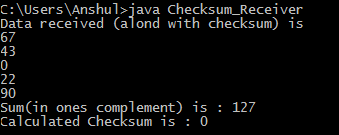Skip to content
Related Articles
Implementing Checksum Using Java
• Last Updated : 02 May, 2018

Checksum is an error-detecting technique that can be applied to message of any length. It is used mostly at the network and transport layers of the TCP/IP protocol suite.
Here, we have considered decimal data that is being sent by the sender to the receiver using socket programming. The number of segments that the data is divided into here depends on the length of data being sent. If the length of data being sent is ‘x’, then the number of segments is also ‘x’, implying that each segment has single data. Here, we basically deal with decimal data. The concept will be consistent for string data as well because each character of the string can be represented by its equivalent ASCII code, hence again leaving us with decimal data.

Prerequisite : Socket Programming in Java | CheckSum

Example :

```At sender side :
Enter data length
4
Enter data to send
67
43
0
22
Checksum Calculated is : 90
Data being sent along with Checksum.....
Thanks for the feedback!!
Message received Successfully!
At receiver side :
Data received (alond with checksum) is
67
43
0
22
90
Sum(in ones complement) is : 127
Calculated Checksum is : 0

Here the checksum calculated at
the receiver side was 0. Hence,
it indicates a successful data transfer.
```

Approach :
At the Sender Side :

1. First, ask for the length of data to send, in order to ascertain the number of segments.
2. Then perform a ones complement of each data being entered simultaneously adding them. This means the sum would not be required to be complemented again.
3. Then send the data along with the computed checksum to the server.
4. Then report the successful transference of the message or otherwise depending on the feedback received from the server.

At the Receiver Side :

1. The receiver waits for data to arrive from the sender.
2. Once the data along with checksum is received from the sender, the receiver complements what is received and simultaneously keeps on adding them.
3. Finally, the receiver complements the above sum, and checks whether the result is a zero or not and reports the same to the sender. A zero would indicate a successful data transfer and anything else would indicate an error in the data that is received.

Finally all connections are closed by both the sides.

Below is the implementation for above approach.
Here, “localhost” is used as the IP to setup the connection with port number 5000 opened for connection. The sender should start running prior and wait for the receiver.

 `// Java code for Checksum_Sender``package` `checksum_sender;`` ` `import` `java.io.*;``import` `java.net.*;``import` `java.util.*;`` ` `public` `class` `Checksum_Sender ``{``    ``// Setting maximum data length``    ``private` `int` `MAX = ``100``;``     ` `    ``// initialize socket and I/O streams``    ``private` `Socket socket = ``null``;``    ``private` `ServerSocket servsock = ``null``;``    ``private` `DataInputStream dis = ``null``;``    ``private` `DataOutputStream dos = ``null``;``     ` `    ``public` `Checksum_Sender(``int` `port) ``throws` `IOException``    ``{``        ``servsock = ``new` `ServerSocket(port);``         ` `        ``// Used to block until a client connects to the server``        ``socket = servsock.accept();``         ` `        ``dis = ``new` `DataInputStream(socket.getInputStream());``        ``dos = ``new` `DataOutputStream(socket.getOutputStream());``         ` `        ``while` `(``true``) ``        ``{``            ``int` `i, l, sum = ``0``, nob;``            ``Scanner sc = ``new` `Scanner(System.in);``            ``System.out.println(``"Enter data length"``);``            ``l = sc.nextInt();``             ` `            ``// Array to hold the data being entered``            ``int` `data[] = ``new` `int``[MAX];``             ` `            ``// Array to hold the complement of each data``            ``int` `c_data[] = ``new` `int``[MAX];``             ` `            ``System.out.println(``"Enter data to send"``);``             ` `            ``for` `(i = ``0``; i < l; i++) ``            ``{``                ``data[i] = sc.nextInt();``                 ` `                ``// Complementing the entered data``                ``// Here we find the number of bits required to represent``                ``// the data, like say 8 requires 1000, i.e 4 bits``                ``nob = (``int``)(Math.floor(Math.log(data[i]) / Math.log(``2``))) + ``1``;``                 ` `                ``// Here we do a XOR of the data with the number 2^n -1,``                ``// where n is the nob calculated in previous step``                ``c_data[i] = ((``1` `<< nob) - ``1``) ^ data[i];``                 ` `                ``// Adding the complemented data and storing in sum``                ``sum += c_data[i];``            ``}``             ` `            ``// The sum(i.e checksum) is also sent along with the data``            ``data[i] = sum;``            ``l += ``1``;``             ` `            ``System.out.println(``"Checksum Calculated is : "` `+ sum);``            ``System.out.println(``"Data being sent along with Checkum....."``);``             ` `            ``// Sends the data length to receiver``            ``dos.writeInt(l);``             ` `            ``// Sends the data one by one to receiver``            ``for` `(``int` `j = ``0``; j < l; j++)``                ``dos.writeInt(data[j]);``             ` `            ``// Displaying appropriate message depending on feedback received``            ``if` `(dis.readUTF().equals(``"success"``)) ``            ``{   ``                ``System.out.println("Thanks ``for` `the feedback!! Message received ``                                   ``Successfully!");``                ``break``;``            ``}``             ` `            ``else` `if` `(dis.readUTF().equals(``"failure"``)) ``            ``{``                ``System.out.println(``"Message was not received successfully!"``);``                ``break``;``            ``}``        ``}``         ` `        ``// Closing all connections``        ``dis.close();``        ``dos.close();``        ``socket.close();``    ``}`` ` `    ``// Driver Method``    ``public` `static` `void` `main(String args[]) ``throws` `IOException``    ``{``        ``Checksum_Sender cs = ``new` `Checksum_Sender(``45678``);``    ``}``}`
 `// Java code for Checksum_Receiver``package` `checksum_sender;`` ` `import` `java.net.*;``import` `java.io.*;``import` `java.util.*;``  ` `public` `class` `Checksum_Receiver {``     ` `    ``// Initialize socket and I/O streams``    ``private` `Socket s = ``null``;``    ``private` `DataInputStream dis = ``null``;``    ``private` `DataOutputStream dos = ``null``;``     ` `    ``// Constructor to put ip address and port``    ``public` `Checksum_Receiver(InetAddress ip,``int` `port)``throws` `IOException``    ``{``         ` `        ``// Opens a socket for connection``        ``s = ``new` `Socket(ip,port);``         ` `        ``dis = ``new` `DataInputStream(s.getInputStream());``        ``dos = ``new` `DataOutputStream(s.getOutputStream());``         ` `        ``while` `(``true``)``        ``{   Scanner sc = ``new` `Scanner(System.in);``            ``int` `i, l, nob, sum = ``0``, chk_sum;``             ` `            ``// Reads the data length sent by sender``            ``l = dis.readInt();``             ` `            ``// Initializes the arrays based on data length received``            ``int` `c_data[] = ``new` `int``[l];``            ``int` `data[] = ``new` `int``[l];``             ` `            ``System.out.println(``"Data received (alond with checksum) is"``);``             ` `            ``for``(i = ``0``; i< data.length; i++)``            ``{   ``                ``// Reading the data being sent one by one``                ``data[i] = dis.readInt();``                ``System.out.println(data[i]);``                 ` `                ``// Complementing the data being received``                ``nob = (``int``)(Math.floor(Math.log(data[i]) / Math.log(``2``))) + ``1``; ``                ``c_data[i] = ((``1` `<< nob) - ``1``) ^ data[i];``                 ` `                ``// Adding the complemented data``                ``sum += c_data[i];``            ``}``            ``System.out.println(``"Sum(in ones complement) is : "``+sum);``             ` `            ``// Complementing the sum``            ``nob = (``int``)(Math.floor(Math.log(sum) / Math.log(``2``))) + ``1``; ``            ``sum = ((``1` `<< nob) - ``1``) ^ sum;``            ``System.out.println(``"Calculated Checksum is : "``+sum);``             ` `            ``// Checking whether final result is 0 or something else``            ``// and sending feedback accordingly ``            ``if``(sum == ``0``)``            ``{   ``                ``dos.writeUTF(``"success"``);``                ``break``;``            ``}     ``            ``else``            ``{   ``                ``dos.writeUTF(``"failure"``);``                ``break``;``            ``}``        ``}``         ` `        ``// Closing all connections``        ``dis.close();``        ``dos.close();``        ``s.close();``    ``}``     ` `    ``// Driver Method``    ``public` `static` `void` `main(String args[])``throws` `IOException``    ``{   ``        ``// Getting ip address on which the receiver is running``        ``// Here, it is "localhost"``        ``InetAddress ip = InetAddress.getLocalHost();``        ``Checksum_Receiver cr = ``new` `Checksum_Receiver(ip,``5000``);``    ``}    ``}`

Output :Attention reader! Don’t stop learning now. Get hold of all the important CS Theory concepts for SDE interviews with the CS Theory Course at a student-friendly price and become industry ready.

My Personal Notes arrow_drop_up